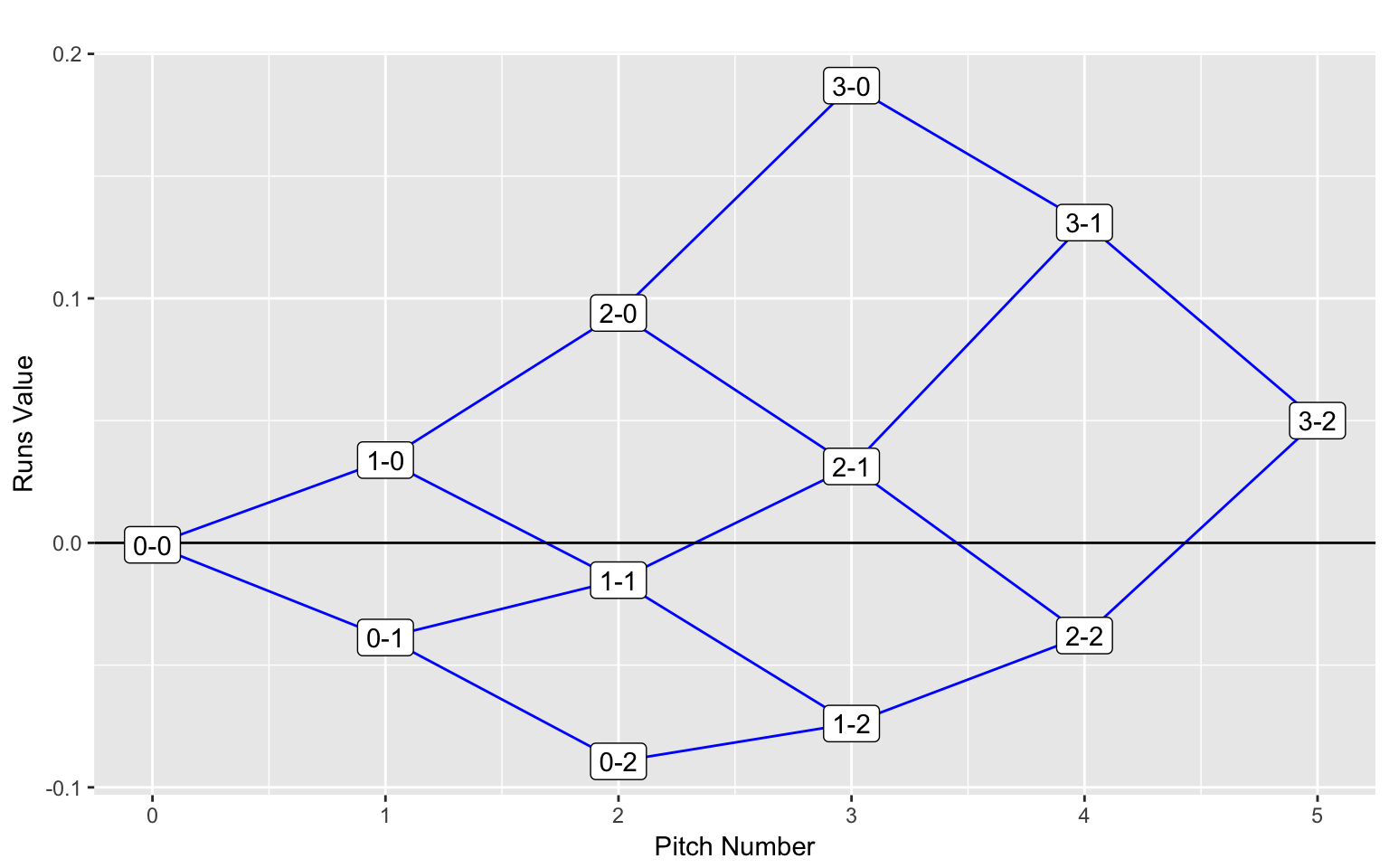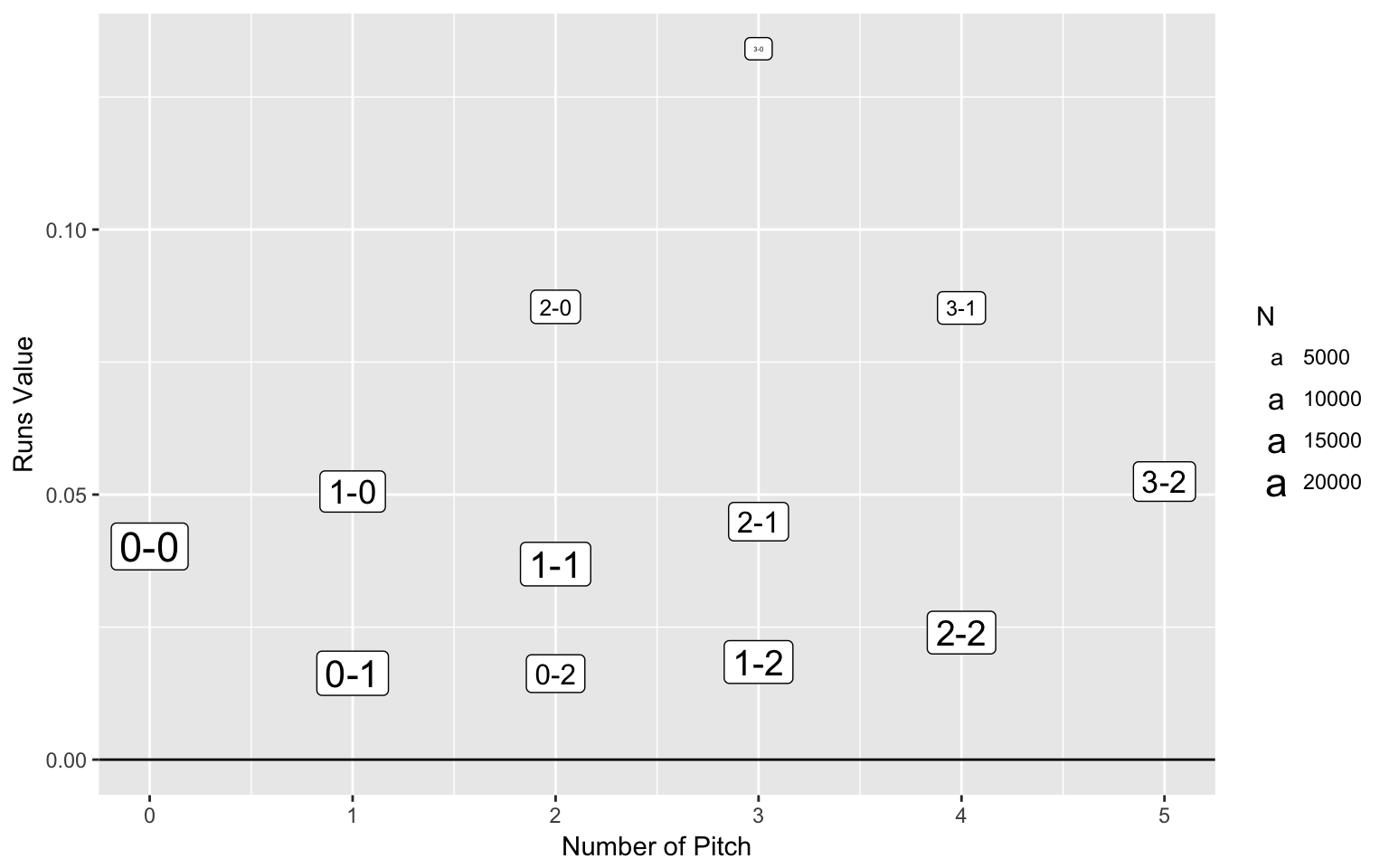### Count Effects

library(readr)
library(ggplot2)
library(dplyr)

#### The Data

Using the Retrosheet play-by-play data for the 2015 season, I found the expected runs in the remainder of the inning for plate appearances that pass through each possible count. I store these expected runs values in the csv file “count2015a.csv”.

I read this file into R – variable name of data frame is d – and show the first few lines.

d <- read_csv("https://bayesball.github.io/VB/data/count2015a.csv")
head(d)
## # A tibble: 6 x 6
##   count strikes balls N.Pitches    Type         Runs
##   <chr>   <int> <int>     <int>   <chr>        <dbl>
## 1   0-0       0     0         0 Neutral -0.000798051
## 2   1-0       0     1         1  Batter  0.033855787
## 3   0-1       1     0         1 Pitcher -0.038708814
## 4   2-0       0     2         2  Batter  0.094016898
## 5   1-1       1     1         2 Pitcher -0.015252581
## 6   0-2       2     0         2 Pitcher -0.089381123

#### The Graph

In this graph, the Pitch Number (variable N.Pitches) is graphed against the Runs Value (variable Runs), using the Count (variable count) as the plotting label.

ggplot(d, aes(N.Pitches, Runs, label=count)) +
geom_point() +
geom_path(data=filter(d, strikes==0),
aes(N.Pitches, Runs), color="blue") +
geom_path(data=filter(d, strikes==1),
aes(N.Pitches, Runs), color="blue") +
geom_path(data=filter(d, strikes==2),
aes(N.Pitches, Runs), color="blue") +
geom_path(data=filter(d, balls==0),
aes(N.Pitches, Runs), color="blue") +
geom_path(data=filter(d, balls==1),
aes(N.Pitches, Runs), color="blue") +
geom_path(data=filter(d, balls==2),
aes(N.Pitches, Runs), color="blue") +
geom_path(data=filter(d, balls==3),
aes(N.Pitches, Runs), color="blue") +
xlab("Pitch Number") +
ylab("Runs Value") +
ggtitle("") +
geom_hline(yintercept=0, color="black") +
geom_label()#### Data

Above we considered the runs value of plate appearances that pass through each possible count. Here we consider the runs values of balls put in play on each possible count. These runs values are found using 2016 Retrosheet play-by-play data. The data is saved in the csv file “count2015b.csv”. We read in this data and save in the variable S.

S <- read_csv("https://bayesball.github.io/VB/data/count2015b.csv")
head(S)
## # A tibble: 6 x 6
##   count       Runs strikes balls N.Pitches     N
##   <chr>      <dbl>   <int> <int>     <int> <int>
## 1   0-0 0.04020994       0     0         0 20668
## 2   0-1 0.01629339       1     0         1 16560
## 3   0-2 0.01622481       2     0         2  8374
## 4   1-0 0.05057780       0     1         1 12366
## 5   1-1 0.03688532       1     1         2 15601
## 6   1-2 0.01841516       2     1         3 14508

#### The Graph

In this graph, the Pitch Number (variable N.Pitches) is graphed against the Runs Value (variable Runs), using the Count (variable count) as the plotting label.

ggplot(S, aes(N.Pitches, Runs, label=count, size=N)) +
xlab("Number of Pitch") +
ylab("Runs Value") +
geom_hline(yintercept=0, color="black") +
geom_label()